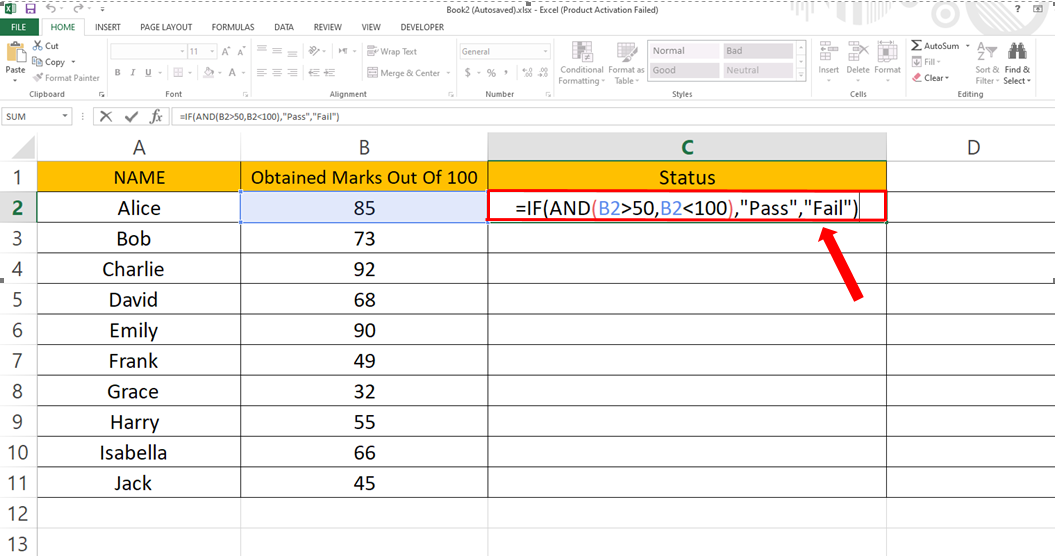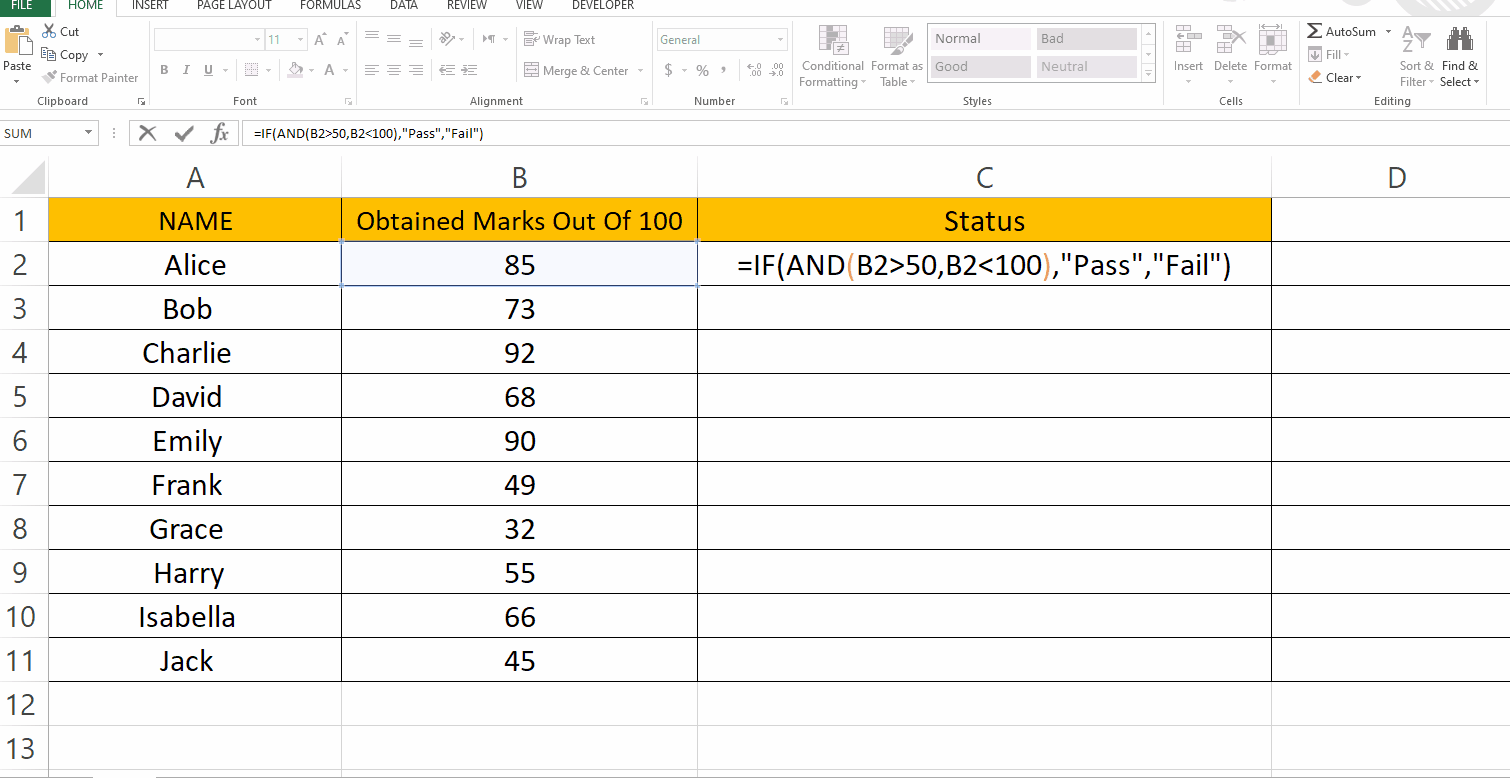# How to use IF with two conditions in ExcelExcel has several built-in functions that allow you to perform operations based on certain conditions. To use these functions, you would typically enter a formula in a cell that includes the function name, the range of cells to be evaluated, and the condition to be tested. The formula would then return the result of the calculation based on the condition that you specified. Here we have a dataset which contains Ten students names and their marks out of 100. In this tutorial we will learn how to use IF statements with two conditions.

### Step 1 – Type in the Formula– For a IF statement with two conditions we will use AND in it.

– Syntax of the formula will be:
=IF(AND(First_Condition, Second_Condition),”Result if value is True”, “Result if value is False”)

– In our case Formula was:
= IF (AND ( B2>50 , B2<100), “Pass”, “Fail”)

– According to this formula if a student scores more than 50 and less than 100 then status will be considered as PASS else FAIL.

### Step 2 – Find status for the rest of the values– After getting the status of the top cell, drag that cell from bottom right to the last cell.

– Status for the rest of the values will appear automatically.